# Lecture 4: The Inverse and the Implicit Function Theorems

Lemma 1: Let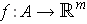be defined and differentiable on a convex open set A. Then for all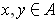, one has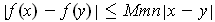where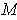is chosen to be an upper bound on all the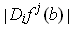for all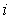,, and all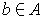.

Proof: Let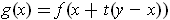. For each, the Mean Value Theorem says that there is a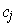between 0 and 1 such that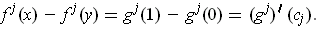By the chain rule,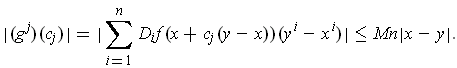So,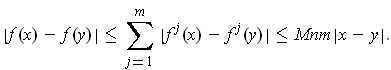Theorem 1: (Inverse Function Theorem) Let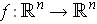be continuously differentiable on an open subset containing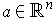. If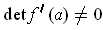, then there are open subsets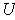containing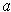and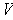containing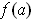such that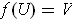and there is an inverse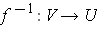which is differentiable with derivative satisfying: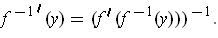Exercise 1: Show that Theorem 1 is true for linear functions.

Proof: (of Theorem 1) By replacingwith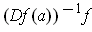, we can assume that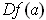is the identity map (Why?).

Apply Lemma 1 to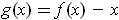: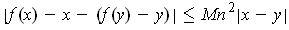for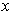and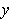in some open rectangle containing. But then,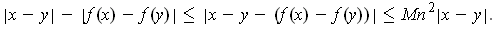Rearranging gives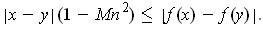Since,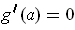and so by choosing the rectangle small enough, we can assume that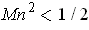and so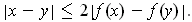In particular, it follows that f is one-to-one when restricted to this rectangle, and the inverse will be continuous if it exists. Replacing the rectangle with a smaller one, we can assume the same is true when f is restricted to the closure of the rectangle. Now the boundary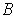of the rectangle is compact and so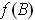is also compact and does not contain. Let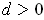be the minimum of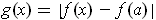for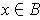. Letbe the set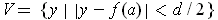.

Now, for every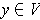, there is at least onein the rectangle with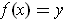. In fact, consider the function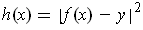. The image of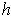under the closure of the rectangle. The minimum of this function does not occur onbecause. So it must occur where the derivative is zero, i.e. one has: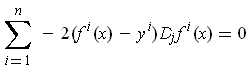for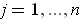. But by taking the rectangle sufficiently small, we can assume that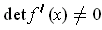for allin the rectangle. But then the only solution of this system of linear equations is.

If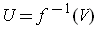, then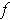maps the open setone-to-one and onto the open set. It remains to check differentiability of the inverse. Sinceis differentiable, one has for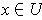,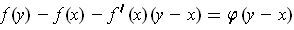where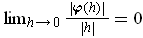. Letting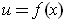and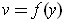, we get after substitution: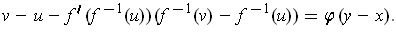Rearranging gives: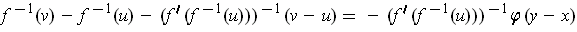It remains to show that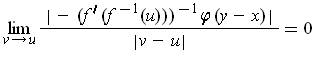Since the derivative is just a linear function, it is enough to show that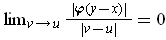. As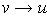, we have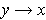because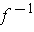is continuous. So,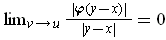. But we know that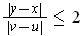So, the limit of the product is zero, as desired.

Note: It follows from the formula for the derivative of the inverse that the inverse is also continuously differentiable.

Corollary 1: (The Implicit Function Theorem) Let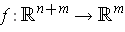be continuously differentiable in an open set containing the point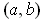which satisfies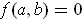. Suppose that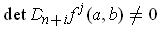. Then there is a continuously differentiable function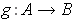mapping an open set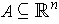containingto an open set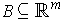containing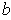such that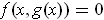for all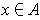.

Proof: We can extendto a function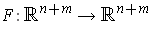by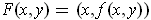and apply the Inverse Function Theorem to get an inverse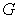defined in an open subset containing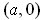and mapping onto an open subset containing. Let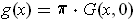where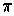is the projection onto the last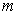coordinates of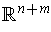. Then one has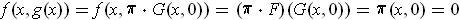.

Exercise 2: Show that the Inverse Function Theorem is a Corollary of the Implicit Function Theorem.